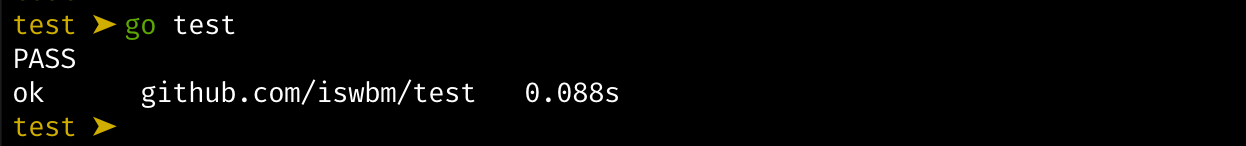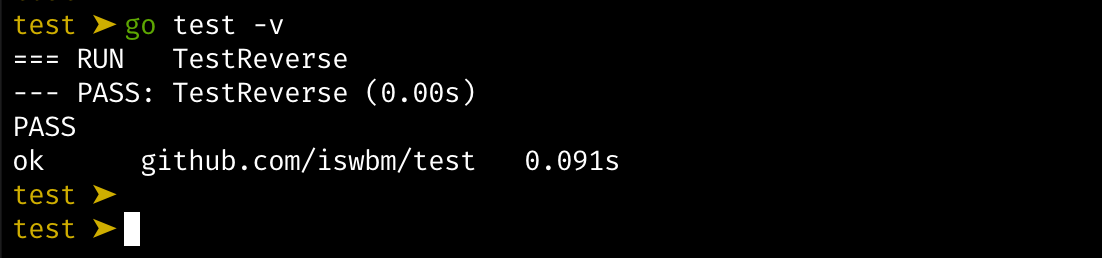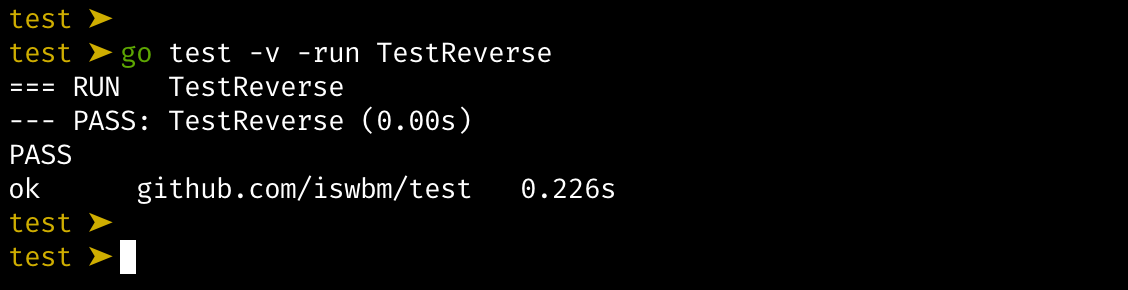# 8.1 测试技巧：单元测试（Unit Test）¶

• 同事修改了某个之前由你编写的函数，但由于同事对这块函数理解上的不足，影响了某个异常场景的处理，你的同事没有测试到，把 bug 流到线上去

• 某个函数的逻辑比较复杂，该函数的改动也很频繁，每一次的改过都要测试非常多的场景，费时费力

## 1. 如何写单元测试¶

```go mod init github.com/iswbm/fuzz
```

```package main

import "fmt"

func Reverse(s string) string {
b := [] byte(s)
for i, j := 0, len(b)-1; i < len(b)/2; i, j = i+1, j-1 {
b[i], b[j] = b[j], b[i]
}
return string(b)
}

func main() {
input := "The quick brown fox jumped over the lazy dog"
rev := Reverse(input)
doubleRev := Reverse(rev)
fmt.Printf("original: %q\n", input)
fmt.Printf("reversed: %q\n", rev)
fmt.Printf("reversed again: %q\n", doubleRev)
}
```

• 给定了三组数据

• 遍历这几组数据，将 tc.in 做为 Reverses 函数的入参执行函数，其返回值跟预期的 tc.want 做对比

• 若不相等，则测试不通过～

```package main

import (
"testing"
)

func TestReverse(t *testing.T) {
testcases := []struct {
in, want string
}{
{"Hello, world", "dlrow ,olleH"},
{" ", " "},
{"!12345", "54321!"},
}
for _, tc := range testcases {
rev := Reverse(tc.in)
if rev != tc.want {
t.Errorf("Reverse: %q, want %q", rev, tc.want)
}
}
}
```

• 单元测试，要导入 testing 包

• 承载测试用例的测试文件，固定以 xxx_test.go（xxx 是原文件名）

• 测试用例函数名称一般命名为 `Test` 加上待测试的方法名。

• 测试用例函数的参数有且只有一个，在这里是 `t *testing.T`

## 2. 执行测试用例¶## 3. 子测试用例¶```package main

import (
"testing"
)

func TestReverse(t *testing.T) {
t.Run("foo", func(t *testing.T) {
testcases := []struct {
in, want string
}{
{"Hello, foo", "oof ,olleH"},
}
for _, tc := range testcases {
rev := Reverse(tc.in)
if rev != tc.want {
t.Errorf("[foo test]Reverse: %q, want %q", rev, tc.want)
}
}
})

t.Run("bar", func(t *testing.T) {
testcases := []struct {
in, want string
}{
{"Hello, bar", "rab ,olleH"},
}
for _, tc := range testcases {
rev := Reverse(tc.in)
if rev != tc.want {
t.Errorf("[bar test] Reverse: %q, want %q", rev, tc.want)
}
}
})
}
```